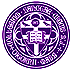Holy Cross Mathematics and Computer Science

# John B. Little

My graduate training, Ph.D. thesis, and my more recent work have all dealt with aspects of the subject known as algebraic geometry. This area of mathematics grew out of the study the geometry of loci defined by polynomial equations, starting with the conic sections, the curves defined by equations 0 = Ax2 + Bxy + Cy2 + Dx + Ey + F. Higher-dimensional objects can also be defined in this way. For instance, here is a Maple plot of the locus

0 = z2 - (16 - x2 - y2) ((x-2)2+y2)/10

Algebraic geometry uses abstract algebra (especially the theory of commutative rings, modules, and so forth) as its primary tool, though parts of the subject also draw on complex analysis, differential geometry, combinatorics, and other parts of mathematics.

Much of my earlier research was devoted to questions about algebraic curves and their Jacobian varieties related to one approach to the Schottky problem of characterizing the Jacobian varieties of curves among all principally polarized abelian varieties.

More recently, I have also become very interested in the new areas of computational algebraic geometry, computer algebra and their applications. The introduction of powerful tools such as Groebner bases and various new versions of resultants for systems of polynomial equations, coupled with the explosive growth in the speed and availability of computational resources, has started to change the face of algebraic geometry. One of the first steps in this theory is a division algorithm for polynomials in several variables.

One interesting area where these techniques have started to have a large influence is the area of computer aided design. For studying geometrically-defined loci such as intersection curves of surfaces, envelopes, offset curves and surfaces, and so forth, computational algebraic geometry provides many concrete tools. Here is an example of a bisector surface of two curves -- the locus of all points P equidistant from (the closest points to P on) a line and a skew parabola in 3D space. (The line and parabola are shown in red.)

Starting from the time of my most recent sabbatical leave (spent at Cornell University), I have also been especially interested in another area where algebraic geometry and its new computational methods are finding some exciting new applications. This area is the field of algebraic coding theory -- specifically the theory of error-correcting codes for reliable information transmission. My interests here are primarily related to the geometric Goppa codes and the construction of algebraic encoding and decoding algorithms for them.

I also enjoy thinking about lots of different areas of mathematics. For example, I enjoy teaching and working on aspects of numerical analysis (as an "amateur"), and I have recently been learning more about Fourier analysis, wavelets, and signal processing.

Here is some information about my recent publications.